﻿

### Existence and multiplicity results for a doubly anisotropic problem with sign-changing nonlinearity

#### Abstract

We consider in this paper the following problem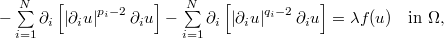$-\sum\limits_{i=1}^{N}\partial _{i}\left[ \left\vert \partial _{i}u\right\vert ^{p_{i}-2}\partial _{i}u\right] -\sum\limits_{i=1}^{N} \partial _{i}\left[ \left\vert \partial _{i}u\right\vert ^{q_{i}-2}\partial _{i}u\right] =\lambda f(u) \text{ \ }\ \text{in } \Omega,\\$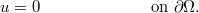$u=0\text{ \ \ \ \ \ \ \ \ \ \ \ \ \ \ \ \ \ \ }\ \text{on }\partial \Omega.$
Where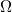$\Omega$ is a bounded regular domain in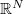$\mathbb{R}^{N}$,$% 1 and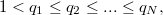$1 we will also assume that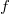$f$ is a continuous function, that have a finite number of zeroes, changing sign between them.

DOI Code: 10.1285/i15900932v39n2p1

Keywords: Anisotropic problem; exitence and mutiplicity; variational methods

Full Text: PDF